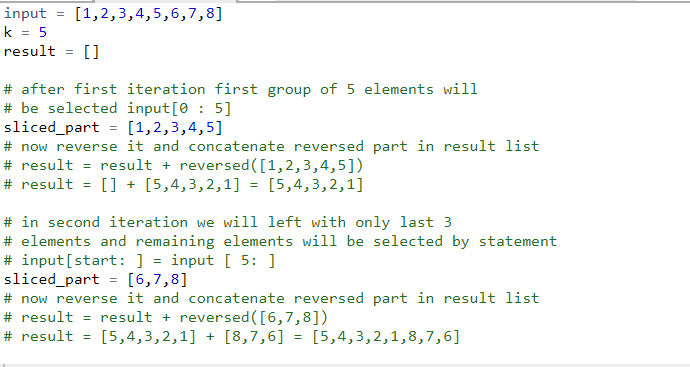Open in App
Not now

# Python Slicing | Reverse an array in groups of given size

• Difficulty Level : Medium
• Last Updated : 11 May, 2020

Given an array, reverse every sub-array formed by consecutive k elements.

Examples:

```Input:
arr = [1, 2, 3, 4, 5, 6, 7, 8, 9]
k = 3
Output:
[3, 2, 1, 6, 5, 4, 9, 8, 7]

Input:
arr = [1, 2, 3, 4, 5, 6, 7, 8]
k = 5
Output:
[5, 4, 3, 2, 1, 8, 7, 6]

Input:
arr = [1, 2, 3, 4, 5, 6]
k = 1
Output:
[1, 2, 3, 4, 5, 6]

Input:
arr = [1, 2, 3, 4, 5, 6, 7, 8]
k = 10
Output:
[8, 7, 6, 5, 4, 3, 2, 1]
```

## Recommended: Please try your approach on {IDE} first, before moving on to the solution.

We have existing solution for this problem please refer Reverse an array in groups of given size link. We can solve this problem quickly in Python using list slicing and reversed() function.Below example will give you better understanding of approach.

Example:`# function to Reverse an array in groups of given size`` ` `def` `reverseGroup(``input``,k):`` ` `    ``# set starting index at 0``    ``start ``=` `0`` ` `    ``# run a while loop len(input)/k times``    ``# because there will be len(input)/k number ``    ``# of groups of size k ``    ``result ``=` `[]``    ``while` `(start<``len``(``input``)):`` ` `           ``# if length of group is less than k``           ``# that means we are left with only last ``           ``# group reverse remaining elements ``           ``if` `len``(``input``[start:])

Output:

```[5, 4, 3, 2, 1, 8, 7, 6]
```

Using Direct Function

 `# function to Reverse an array in groups of given size``  ` `def` `reverseGroup(a, k):`` ` `   ``# take an empty list``   ``res ``=` `[]`` ` `   ``# iterate over the list with increment of ``   ``# k times in each iteration``   ``for` `i ``in` `range``(``0``, ``len``(a), k):``      ` `       ``# reverse the list in each iteration over ``       ``# span of k elements using extend``       ``res.extend((a[i:i ``+` `k])[::``-``1``])``   ``print``(res)``  ` `# Driver program``if` `__name__ ``=``=` `"__main__"``:``    ``input` `=` `[``1``, ``2``, ``3``, ``4``, ``5``, ``6``, ``7``, ``8``]``    ``k ``=` `5``    ``reverseGroup(``input``, k)`

Output:

```[5, 4, 3, 2, 1, 8, 7, 6]
```

My Personal Notes arrow_drop_up